# NCERT Solutions for Class 10 Maths Exercise 11.2## myCBSEguide App

Complete Guide for CBSE Students

NCERT Solutions, NCERT Exemplars, Revison Notes, Free Videos, CBSE Papers, MCQ Tests & more.

NCERT solutions for Maths Constructions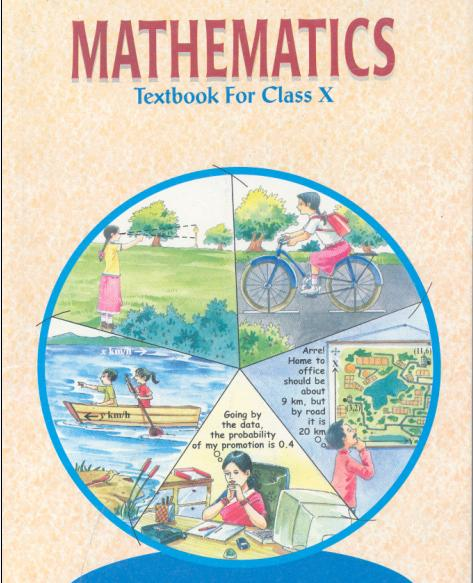## NCERT Solutions for Class 10 Maths Constructions

Exercise 11.2

In each of the following, give the justification of the construction also:

###### 1. Draw a circle of radius 6 cm. From a point 10 cm away from its centre, construct the pair of tangents to the circle and measure their lengths.

Ans. Given: A circle whose centre is O and radius is 6 cm and a point P is 10 cm away from its centre.

To construct: To construct the pair of tangents to the circle and measure their lengths.

Steps of Construction: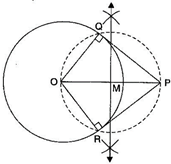(a) Join PO and bisect it. Let M be the mid-point of PO.

(b) Taking M as centre and MO as radius, draw a circle. Let it intersects the given circle at the points Q and R.

(c) Join PQ and PR.

Then PQ and PR are the required two tangents.

By measurement, PQ = PR = 8 cm

Justification: Join OQ and OR.

Since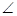OQP andORP are the angles in semicircles.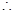OQP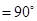=ORP

Also, since OQ, OR are radii of the circle, PQ and PR will be the tangents to the circle at Q and R respectively.We may see that the circle with OP as diameter increases the given circle in two points. Therefore, only two tangents can be draw.

NCERT Solutions for Class 10 Maths Exercise 11.2

###### 2. Construct a tangent to a circle of radius 4 cm from a point on the concentric circle of radius 6 cm and measure its length. Also verify the measurement by actual calculation.

Ans. To construct: To construct a tangent to a circle of radius 4 cm from a point on the concentric circle of radius 6 cm and measure its lengths. Also to verify the measurements by actual calculation.

Steps of Construction: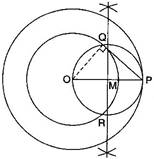(a) Join PO and bisect it. Let M be the mid-point of PO.

(b) Taking M as centre and MO as radius, draw a circle. Let it intersects the given circle at the point Q and R.

(c) Join PQ.

Then PQ is the required tangent.

By measurement, PQ = 4.5 cm

By actual calculation,

PQ =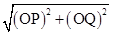=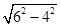=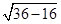=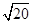= 4.47 cm

Justification: Join OQ. ThenPQO is an angle in the semicircle and therefore,PQO =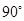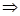PQ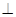OQ

Since, OQ is a radius of the given circle, PQ has to be a tangent to the circle.

NCERT Solutions for Class 10 Maths Exercise 11.2

###### 3. Draw a circle of radius 3 cm. Take two points P and Q on one of its extended diameter each at a distance of 7 cm from its centre. Draw tangents to the circle from these two points P and Q.

Ans. To construct: A circle of radius 3 cm and take two points P and Q on one of its extended diameter each at a distance of 7 cm from its centre and then draw tangents to the circle from these two points P and Q.

Steps of Construction: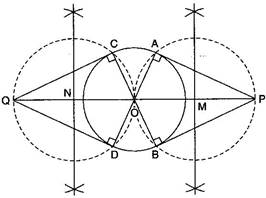(a) Bisect PO. Let M be the mid-point of PO.

(b) Taking M as centre and MO as radius, draw a circle. Let it intersects the given circle at the points A and B.

(c) Join PA and PB.

Then PA and PB are the required two tangents.

(d) Bisect QO. Let N be the mid-point of QO.

(e) Taking N as centre and NO as radius, draw a circle. Let it intersects the given circle at the points C and D.

(f) Join QC and QD.

Then QC and QD are the required two tangents.

Justification: Join OA and OB.

ThenPAO is an angle in the semicircle and thereforePAO =.PAOA

Since OA is a radius of the given circle, PA has to be a tangent to the circle. Similarly, PB is also a tangent to the circle.

Again join OC and OD.

ThenQCO is an angle in the semicircle and thereforeQCO =.

Since OC is a radius of the given circle, QC has to be a tangent to the circle. Similarly, QD is also a tangent to the circle.

NCERT Solutions for Class 10 Maths Exercise 11.2

###### 4. Draw a pair of tangents to a circle of radius 5 cm which are inclined to each other at an angle of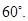Ans. To construct: A pair of tangents to a circle of radius 5 cm which are inclined to each other at an angle ofSteps of Construction: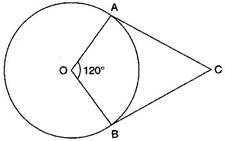(a) Draw a circle of radius 5 cm with centre O.

(b) Draw an angle AOB of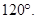(c) At A and B, drawangles which meet at C.

Then AC and BC are the required tangents which are inclined to each other at an angle ofJustification: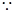OAC =and OA is a radius.

[By construction]AC is a tangent to the circle.OBC =and OB is a radius.

[By construction]BC is a tangent to the circle.AOB +OAC +OBC +ACB =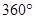[Angle sum property of a quadrilateral]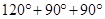+ACB =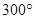+ACB =ACB =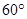NCERT Solutions for Class 10 Maths Exercise 11.2

###### 5. Draw a line segment AB of length 8 cm. Taking A as centre, draw a circle of radius 4 cm and taking B as centre, draw another circle of radius 3 cm. Construct tangents to each circle from the centre of the other circle.

Ans. To construct: A line segment of length 8 cm and taking A as centre, to draw a circle of radius 4 cm and taking B as centre, draw another circle of radius 3 cm. Also, to construct tangents to each circle from the centre to the other circle.

Steps of Construction: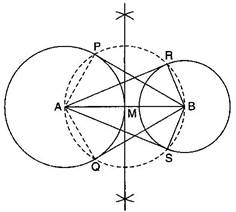(a) Bisect BA. Let M be the mid-point of BA.

(b) Taking M as centre and MA as radius, draw a circle. Let it intersects the given circle at the points P and Q.

(c) Join BP and BQ.

Then, BP and BQ are the required two tangents from B to the circle with centre A.

(d) Again, Let M be the mid-point of AB.

(e) Taking M as centre and MB as radius, draw a circle. Let it intersects the given circle at the points R and S.

(f) Join AR and AS.

Then, AR and AS are the required two tangents from A to the circle with centre B.

Justification: Join BP and BQ.

ThenAPB being an angle in the semicircle is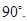BPAP

Since AP is a radius of the circle with centre A, BP has to be a tangent to a circle with centre A. Similarly, BQ is also a tangent to the circle with centre A.

Again join AR and AS.

ThenARB being an angle in the semicircle isARBR

Since BR is a radius of the circle with centre B, AR has to be a tangent to a circle with centre B. Similarly, AS is also a tangent to the circle with centre B.

NCERT Solutions for Class 10 Maths Exercise 11.2

###### 6. Let ABC be a right triangle in which AB = 6 cm, BC = 8 cm andB =BD is the perpendicular from B on AC. The circle through B, C, D is drawn. Construct the tangents from A to this circle.

Ans. To construct: A right triangle ABC with AB = 6 cm, BC = 8 cm andB =BD is the perpendicular from B on AC and the tangents from A to this circle.

Steps of Construction: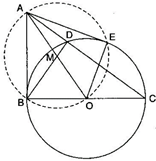(a) Draw a right triangle ABC with AB = 6 cm, BC = 8 cm andB =Also, draw perpendicular BD on AC.

(b) Join AO and bisect it at M (here O is the centre of circle through B, C, D).

(c) Taking M as centre and MA as radius, draw a circle. Let it intersects the given circle at the points B and E.

(d) Join AB and AE.

Then AB and AE are the required two tangents.

Justification: Join OE.

Then,AEO is an angle in the semicircle.AEO =AEOE

Since OE is a radius of the given circle, AE has to be a tangent to the circle. Similarly, AB is also a tangent to the circle.

NCERT Solutions for Class 10 Maths Exercise 11.2

###### 7. Draw a circle with the help of a bangle. Take a point outside the circle. Construct the pair of tangents from this point to the circle.

Ans. To construct: A circle with the help of a bangle. Take a point outside the circle. Construct the pair of tangents from this point to the circle.

Steps of Construction: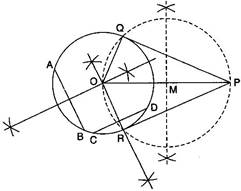(a) Draw a circle with the help of a bangle.

(b) Take two non-parallel chords AB and CD of this circle.

(c) Draw the perpendicular bisectors of AB and CD. Let these intersect at O. Then O is the centre of the circle draw.

(d) Take a point P outside the circle.

(e) Join PO and bisect it. Let M be the mid-point of PO.

(f) Taking M as centre and MO as radius, draw a circle. Let it intersects the given circle at the points Q and R.

(g) Join PQ and PR.

Then PQ and PR are the required two tangents.

Justification: Join OQ and OR.

Then,PQO is an angle in the semicircle.PQO =PQOQ

Since OQ is a radius of the given circle, PQ has to be a tangent to the circle. Similarly, PR is also a tangent to the circle.

## NCERT Solutions for Class 10 Maths Exercise 11.2

NCERT Solutions for Class 10 Maths Exercise 11.2 PDF (Download) Free from myCBSEguide app and myCBSEguide website. Ncert solution class 10 Maths includes text book solutions from Mathematics Book. NCERT Solutions for CBSE Class 10 Maths have total 15 chapters. 10 Maths NCERT Solutions in PDF for free Download on our website. Ncert Maths class 10 solutions PDF and Maths ncert class 10 PDF solutions with latest modifications and as per the latest CBSE syllabus are only available in myCBSEguide.

## CBSE app for Class 10

To download NCERT Solutions for Class 10 Maths, Computer Science, Home Science,Hindi ,English, Social Science do check myCBSEguide app or website. myCBSEguide provides sample papers with solution, test papers for chapter-wise practice, NCERT solutions, NCERT Exemplar solutions, quick revision notes for ready reference, CBSE guess papers and CBSE important question papers. Sample Paper all are made available through the best app for CBSE## Test Generator

Create Papers with your Name & Logo

### 14 thoughts on “NCERT Solutions for Class 10 Maths Exercise 11.2”

1. This site is very good and helpful
Many poor people who could not afford tuitions can see their doubts here .

2. How can they afford internet? Sarvagay Sanmotra

it helped me too score good marks…

4. It helped me a lot…………thnxxx

5. Nice i loved it!!

6. I love it

7. It is helped
It is good score my marks
????????

8. It’s very useful site for me …….Thñx…

9. It helped me a lot in clearing my doubts. Thanxx??